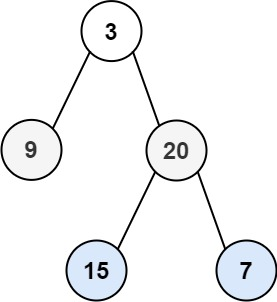## C# || How To Traverse Binary Tree Level Order Using C#The following is a module with functions which demonstrates how to traverse binary tree level order using C#.

1. Level Order – Problem Statement

Given the root of a binary tree, return the level order traversal of its nodes’ values. (i.e., from left to right, level by level).

Example 1:``` Input: root = [3,9,20,null,null,15,7] Output: [,[9,20],[15,7]] ```

Example 2:

``` Input: root =  Output: [] ```

Example 3:

``` Input: root = [] Output: [] ```

2. Level Order – Solution

The following is a solution which demonstrates how to traverse binary tree level order.

This solution uses Breadth First Search to explore items at each level.

``` 2. Level Order - Solution C# // ============================================================================ // Author: Kenneth Perkins // Date: Oct 29, 2021 // Taken From: http://programmingnotes.org/ // File: Solution.cs // Description: Determines how to traverse a tree level order // ============================================================================ /** * Definition for a binary tree node. * public class TreeNode { * public int val; * public TreeNode left; * public TreeNode right; * public TreeNode(int val=0, TreeNode left=null, TreeNode right=null) { * this.val = val; * this.left = left; * this.right = right; * } * } */ public class Solution { public IList<IList<int>> LevelOrder(TreeNode root) { var result = new List<IList<int>>(); var queue = new Queue<TreeNode>(); if (root != null) { queue.Enqueue(root); } while (queue.Count > 0) { var level = new List<int>(); // Loop through items in the queue for (var itemCount = queue.Count; itemCount > 0; --itemCount) { var current = queue.Peek(); queue.Dequeue(); // Add children to the queue if they exist if (current.left != null) { queue.Enqueue(current.left); } if (current.right != null) { queue.Enqueue(current.right); } // Add current value to the level level.Add(current.val); } // Add items on this level to the result result.Add(level); } return result; } }// http://programmingnotes.org/ 1234567891011121314151617181920212223242526272829303132333435363738394041424344454647484950515253545556 // ============================================================================//    Author: Kenneth Perkins//    Date:   Oct 29, 2021//    Taken From: http://programmingnotes.org///    File:  Solution.cs//    Description: Determines how to traverse a tree level order// ============================================================================/** * Definition for a binary tree node. * public class TreeNode { *     public int val; *     public TreeNode left; *     public TreeNode right; *     public TreeNode(int val=0, TreeNode left=null, TreeNode right=null) { *         this.val = val; *         this.left = left; *         this.right = right; *     } * } */public class Solution {    public IList<IList<int>> LevelOrder(TreeNode root) {        var result = new List<IList<int>>();        var queue = new Queue<TreeNode>();         if (root != null) {            queue.Enqueue(root);        }         while (queue.Count > 0) {            var level = new List<int>();             // Loop through items in the queue            for (var itemCount = queue.Count; itemCount > 0; --itemCount) {                var current = queue.Peek();                queue.Dequeue();                 // Add children to the queue if they exist                if (current.left != null) {                    queue.Enqueue(current.left);                }                if (current.right != null) {                    queue.Enqueue(current.right);                }                 // Add current value to the level                level.Add(current.val);            }             // Add items on this level to the result            result.Add(level);        }         return result;    }}// http://programmingnotes.org/ ```

QUICK NOTES:
The highlighted lines are sections of interest to look out for.

The code is heavily commented, so no further insight is necessary. If you have any questions, feel free to leave a comment below.

Once compiled, you should get this as your output for the example cases:

``` [,[9,20],[15,7]] [] [] ```